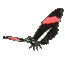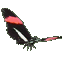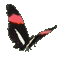Graphs of Power Functions     Math Help     Game Tips:

- The relations y=x2, y=x3, and y=x4 are examples of power functions.

- A 'variable base raised to a constant exponent' = (variable)(constant) is a 'Power function'.
- In general, a 'Power function' can be represented by a formula such as y=xn.

- The relations y=3x2, y=4x6, and y=2x3 are examples of power functions with a front multiplier.
- In general, a 'Constant times a Power function' can be represented by a formula such as y=kxn.

- In this game, 'k' is an integer from -3 to 3 and 'n' is an integer from 2 to 5.

- Notice y=x2 has graph points such as (-1,1), (0,0), (1,1), and (2,4)... ,
while a relation such as y=2x3 has graph points such as (-1,-2), (0,0), (1,2), and (2,16)... .

- There are many applications of power functions. For example, the area of a circle
is a function of (radius)2, and the volume of a cube is a function of (side)3.

- Your Game Score is reduced by the number of butterfly hits.

- To slow the game speed repeat tap/click on the word Slider.
- To increase the game speed repeat tap/click on the word Math.
- Speed can also be adjusted with a keyboard's - and + keys.

- Refresh/Reload the web page to restart the game.